Basics of Derivative Pricing and Valuation | IFT World
IFT Notes for Level I CFA® Program
IFT Notes for Level I CFA® Program

# Part 2

### 4.1 Pricing and Valuation of Forward Commitments

Pricing and Valuation of Forward Contracts

Let us take an example. Assume Leo owns a share of GE, whose spot price today (S0) is $100. Rachel enters into a forward contract with Leo today to buy a share of GE at$101 after 1 month.

The terms, parties and ways to settle the contract are described below:

• $101 is the forward price; the contract is for a period of 1 month. • Neither Rachel nor Leo pays any money to each other at t = 0 when they enter into the contract. • Rachel is the long party and Leo is the short party. • When the contract expires after 1 month, the forward contract can be settled in two ways: 1) Rachel pays$101 to Leo and receives the share in return; 2) if GE trades at $103 after 1 month, then Leo pays$2 (the difference between the agreed-upon forward price and the current stock price) to Rachel.
• There is a risk of default in forward contracts. If the stock price increases to $103, Rachel faces the risk that Leo fails to deliver the share as agreed. Conversely, if the stock price decreases to$99, Leo faces the risk that Rachel does not buy the share as agreed upon.
• Is there an alternative way for Rachel to buy this asset? Yes, to wait until time T and buy the asset at its then spot price S The drawback is that one cannot be sure what the price of the asset will be then; it may be more or less.

The above example is shown in a generic way in the diagram below. It shows the transactions from a buyer’s perspective at times t = 0 when the contract is initiated and time t = T, when the contract expires.

Interpreting the notation and key features of a forward contract:

• Forward price: It is denoted by F(T) where subscript 0 indicates the price that is agreed upon at time t = 0 and T indicates the contract ends at time T.
• Spot price: It is denoted by St At t = 0, spot price is S0. When the forward contract expires at t = T, the spot price is ST. At any time during the life of the contract, it is denoted by St
• Value of the contract at time t is denoted by Vt(T).
• The price to be paid at time T when the contract expires is fixed at time t = 0.
• No money exchanges hands at time t = 0 i.e. no party pays anything to the other at t = 0.

The forward price agreed at the initiation date of the contract is the spot rate compounded at the risk-free rate over the life of the contract.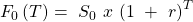Assuming a risk-free rate of 10%, spot price of , and time period of 1 month, we calculate the forward price as:

T =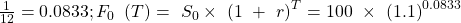= 101

Why is the forward price equal to the spot rate compounded at the risk-free rate over the contract period?

In theory, if Rachel did not enter into the forward contract, then she could invest $100 for period T in a risk-free asset and earn 10% or$101 after 1 month. So, the forward contract must earn the risk-free rate as an excess return will result in an arbitrage opportunity.

Value of a forward contract at initiation

Value of a forward contract at initiation = 0

The value of a forward contract at initiation is zero because neither party pays any money to the other. There is no value to either party.

• At the end of the contract, Leo as the short party, is obligated to sell the asset to the buyer (Rachel) for $101. • Given a spot price of$100, a risk-free rate of 10% and a forward price of $101, there is no advantage to either party at t = 0. The forward price is such that no party can earn an arbitrage profit. Value at expiration for the long party: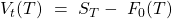where: ST= spot price of the underlying F0(T)= forward price agreed in the contract The value of a forward contract is positive to the long party if ST > F0(T) and negative if ST<F0(T) at expiration. Value at expiration for the short party: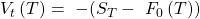The value of a forward contract is positive to the short party if F0(T) > ST and negative if F0(T) < ST at expiration. If the spot price at time T is 105, then Rachel (long) gets the asset by paying 101 and can immediately sell it for 105 at the then market price. Value to the long =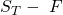= 105 – 101 = 4. ## 5. Pricing and Valuation of Forward Contracts: Between Initiation and Expiration; Forward Rate Agreements Value of a forward contract during the life of the contract Value during the life of the contract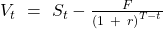This is the difference between the spot price at time t, and the present value of forward price for the remaining life of the contract. In our example, at t = 15 days, if St= 106, then Vt =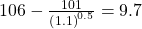Since the price has gone up, the value to the long party is positive. Instructor’s Note As market conditions change, only the value of a forward contract changes. Its price does not change as it is fixed at contract initiation. Forward Price with Benefits and Costs Now, let us consider an asset with benefits and costs. How is the forward price for such an asset calculated? The forward price of an asset with benefits and/or costs is the spot price compounded at the risk-free rate over the life of the contract minus the future value of those benefits and costs.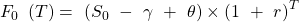It can be rewritten as: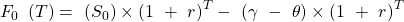where γ = present value of the benefit. It is subtracted from the spot price because if you own the asset, you receive any benefits associated with the asset, during the life of the contract. θ = present value of costs incurred on the asset during the life of the contract. These costs make it more expensive to hold the asset and hence increase the forward price. In the previous example, assume$10 is the present value of benefits from the asset and $20 is the present value of costs associated with holding the asset. The forward price at time 0 when Rachel enters into the contract is: F(T)=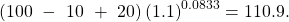The value of a forward contract is the spot price of the underlying asset minus the present value of the forward price. The value of the contract at time t is given by the expression below: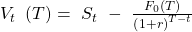(without benefits and costs)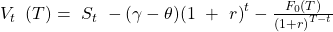(with benefits and costs) In words, the value of the contract is the spot price minus the net benefit for the remaining period minus the present value of the forward price. Note that (γ – θ) is the net benefit. Instructor’s Note The forward price can be lower than the spot price though it is not common.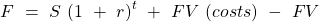(benefits) When the future value of benefits is higher than the future value of costs and the compounded spot price, then the forward price is lower than the spot price. If a commodity is in short supply, then its non-monetary benefits/convenience yield may be higher. ### 5.1 A Word about Forward Contracts on Interest Rates So far, the forward contracts we have seen had an asset as the underlying. But, some forward contracts which have an interest rate as the underlying are called forward rate agreements. FRAs allow us to lock in a rate today for a loan in the future and act as a hedge against interest rate risk. They are forward contracts that allow participants to make a known interest payment at a later date and receive in return an unknown interest payment. The payoffs on an FRA are determined by market interest rates at expiration: • If reference rate at FRA expiration is greater than the FRA rate, then the long benefits. • If reference rate at FRA expiration is lower than the FRA rate, then the short benefits. Example of an FRA The exhibit below shows going long a 30-day FRA in which the underlying is 90-day Libor. Source: CFA Program Curriculum, Basics of Derivative Pricing and Valuation Interpretation: • FRAs are usually based on LIBOR (London Interbank Offered Rate). The FRA described above is a 30-day FRA in which the underlying is the 90-day LIBOR. • Assume the time now is t = 0 and you need to borrow$100 million after 30 days. You are concerned that the interest rate represented by LIBOR might increase between now and 30 days, and would like to lock in a rate available now of 5%. This is the rate that will be applicable on the loan 30 days later. This removes any uncertainty regarding the interest payment on the loan, as it is fixed today. The rate is for a period, in this case for 90 days. You enter into an agreement today to borrow \$100 million 30 days later at a rate of 5% for 90 days.
• After 90 days, if the rate increases to 6%, then you benefit as your outflow is based on 5%, but you receive an inflow based on 6%. However, you lose if the rate decreases to 4% as the outflow will be higher than what you receive.
• Are FRAs of interest only to parties that borrow money? No, even parties that lend money engage in FRAs to lock in an interest rate if they believe the rates will go down in the future.

Synthetic FRA: Instead of engaging in a real FRA, a synthetic FRA can be constructed by lending a 30-day Eurodollar time deposit and buying a 120-day Eurodollar time deposit.

Instructor’s Note

A quick summary of the concepts discussed in earlier readings.

1. The rate on a zero-coupon bond is also a spot rate.
2. Term structure of interest rates: interest rates on loans of different maturities. For example, the 30-day rate is 6%, 60-day rate is 7% and so on.